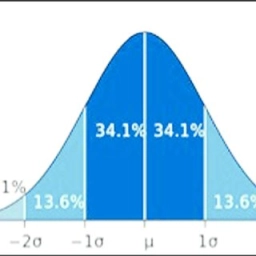# Suppose 8

Suppose the scores on a test have a normal distribution with X=74 and a standard deviation of s=18.

What percentage of students have scores higher than 90?
What percentage of students have scores between 70 and 85?

Twenty percent of the students do better than what they score on the exam. Forty-two percent of the students do worse than they scored on the exam.

a =  18.7 %
b =  31.74 %
c =  89.138
d =  69.41

### Step-by-step explanation:Did you find an error or inaccuracy? Feel free to write us. Thank you!

Tips for related online calculators
Looking for help with calculating arithmetic mean?
Looking for a statistical calculator?
Looking for a standard deviation calculator?